# NCERT Solutions For Class 12 Chemistry chapter 7-The P Block Elements

## NCERT Solutions for Class 12 Chemistry

NCERT Solutions for class-12 Chemistry Chapter 7 The P Block Elements is prepared by our senior and renowned teachers of Physics Wallah primary focus while solving these questions of class-12 in NCERT textbook, also do read theory of this Chapter 7 The P Block Elements while going before solving the NCERT questions. Our Physics Wallah team Prepared Other Subjects NCERT Solutions for class 12.

### Answer the following Questions.

Question1. Why are pentahalides more covalent than trihalides?

Solution :
In pentahalides, the oxidation state is +5 and in trihalides, the oxidation state is +3. Since the metal ion with a high charge has more polarizing power, pentahalides are more covalent than trihalides.

Question2. Why is BiH3 the strongest reducing agent amongst all the hydrides of Group 15 elements?

Solution :
As we move down a group, the atomic size increases and the stability of the hydrides of group 15 elements decreases. Since the stability of hydrides decreases on moving from NH3 to BiH3, the reducing character of the hydrides increases on moving from NH3 to BiH3.

Question3. Why is N2 less reactive at room temperature?

Solution :
The two N atoms in N2 are bonded to each other by very strong triple covalent bonds. The bond dissociation energy of this bond is very high. As a result, N2 is less reactive at room temperature.

Question4. Mention the conditions required to maximise the yield of ammonia.

Solution :
Ammonia is prepared using the Haber’s process. The yield of ammonia can be maximized under the following conditions:

(i) High pressure (∼ 200 atm)

(ii) A temperature of ∼700 K

(iii) Use of a catalyst such as iron oxide mixed with small amounts of K2O and Al2O3

Question5. How does ammonia react with a solution of Cu2+?

Solution :
NH3 acts as a Lewis base. It donates its electron pair and forms a linkage with metal ion.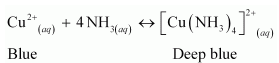Question6. What is the covalence of nitrogen in N2O5?

Solution :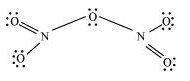From the structure of N2O5, it is evident that the covalence of nitrogen is 4.

Question7. Bond angle in PH+4 is higher than that in PH3. Why?

Solution :
In PH3, P is sp3 hybridized. Three orbitals are involved in bonding with three hydrogen atoms and the fourth one contains a lone pair. As lone pair-bond pair repulsion is stronger than bond pair-bond pair repulsion, the tetrahedral shape associated with sp3 bonding is changed to pyramidal. PH3 combines with a proton to form PH+4 in which the lone pair is absent. Due to the absence of lone pair in PH+4, there is no lone pair-bond pair repulsion. Hence, the bond angle in PH+4 is higher than the bond angle in PH3.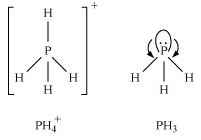Question8. What happens when white phosphorus is heated with concentrated NaOH solution in an inert atmosphere of CO2?

Solution :
White phosphorous dissolves in boiling NaOH solution (in a CO2 atmosphere) to give phosphine, PH3.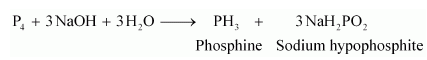Question9. What happens when PCl5 is heated?

Solution :
All the bonds that are present in PCl5 are not similar. It has three equatorial and two axial bonds. The equatorial bonds are stronger than the axial ones. Therefore, when PCl5 is heated strongly, it decomposes to form PCl3.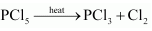Question10. Write a balanced equation for the hydrolytic reaction of PCl5 in heavy water.

Solution :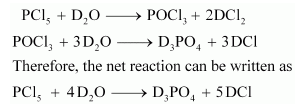Question11. What is the basicity of H3PO4?

Solution :
H3PO4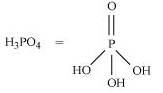Since there are three OH groups present in H3PO4, its basicity is three i.e., it is a tribasic acid.

Question12. What happens when H3PO3 is heated?

Solution :
H3PO3, on heating, undergoes disproportionation reaction to form PH3 and H3PO4. The oxidation numbers of P in H3PO3, PH3, and H3PO4 are +3, −3, and +5 respectively. As the oxidation number of the same element is decreasing and increasing during a particular reaction, the reaction is a disproportionation reaction.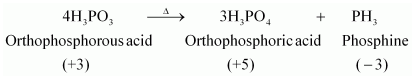Question13. List the important sources of sulphur.

Solution :
Sulphur mainly exists in combined form in the earth’s crust primarily as sulphates [gypsum (CaSO4.2H2O), Epsom salt (MgSO4.7H2O), baryte (BaSO4)] and sulphides [(galena (PbS), zinc blends (ZnS), copper pyrites (CuFeS2)].

Question14. Write the order of thermal stability of the hydrides of Group 16 elements.

Solution :
The thermal stability of hydrides decreases on moving down the group. This is due to a decrease in the bond dissociation enthalpy (H−E) of hydrides on moving down the group.

Therefore,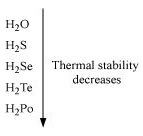Question15. Why is H2O a liquid and H2S a gas?

Solution :
H2O has oxygen as the central atom. Oxygen has smaller size and higher electronegativity as compared to sulphur. Therefore, there is extensive hydrogen bonding in H2O, which is absent in H2S. Molecules of H2S are held together only by weak van der Waal’s forces of attraction.

Hence, H2O exists as a liquid while H2S as a gas.

Question16. Which of the following does not react with oxygen directly?

Zn, Ti, Pt, Fe

Solution :
Pt is a noble metal and does not react very easily. All other elements, Zn, Ti, Fe, are quite reactive. Hence, oxygen does not react with platinum (Pt) directly.

Question17. Complete the following reactions:

(i) C2H4 + O2

(ii) 4Al + 3O2

Solution :
(i)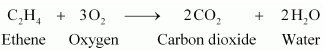(ii)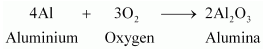Question18. Why does O3 act as a powerful oxidising agent?

Solution :
Ozone is not a very stable compound under normal conditions and decomposes readily on heating to give a molecule of oxygen and nascent oxygen. Nascent oxygen, being a free radical, is very reactive.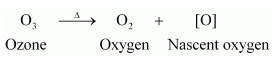Therefore, ozone acts as a powerful oxidising agent.

Question19. How is O3 estimated quantitatively?

Solution :
Quantitatively, ozone can be estimated with the help of potassium iodide. When ozone is made to react with potassium iodide solution buffered with a borate buffer (pH 9.2), iodine is liberated. This liberated iodine can be titrated against a standard solution of sodium thiosulphate using starch as an indicator. The reactions involved in the process are given below.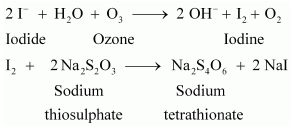Question20. What happens when sulphur dioxide is passed through an aqueous solution of Fe(III) salt?

Solution :
SO2 acts as a reducing agent when passed through an aqueous solution containing Fe(III) salt. It reduces Fe(III) to Fe(II) i.e., ferric ions to ferrous ions.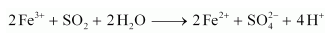Question21. Comment on the nature of two S−O bonds formed in SO2 molecule. Are the two S−O bonds in this molecule equal?

Solution :
The electronic configuration of S is 1s2 2s2 2p6 3s2 3p4.

During the formation of SO2, one electron from 3p orbital goes to the 3d orbital and S undergoes sp2 hybridization. Two of these orbitals form sigma bonds with two oxygen atoms and the third contains a lone pair. p-orbital and d-orbital contain an unpaired electron each. One of these electrons forms pπ- pπ bond with one oxygen atom and the other forms pπ- dπ bond with the other oxygen. This is the reason SO2 has a bent structure. Also, it is a resonance hybrid of structures I and II.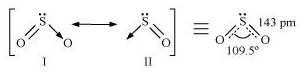Both S−O bonds are equal in length (143 pm) and have a multiple bond character.

Question22. How is the presence of SO2 detected?

Solution :
SO2 is a colourless and pungent smelling gas.

It can be detected with the help of potassium permanganate solution. When SO2 is passed through an acidified potassium permanganate solution, it decolonizes the solution as it reduces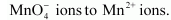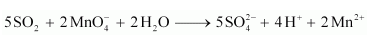Question23. Mention three areas in which H2SO4 plays an important role.

Solution :
Sulphuric acid is an important industrial chemical and is used for a lot of purposes. Some important uses of sulphuric acid are given below.

• It is used in fertilizer industry. It is used to make various fertilizers such as ammonium sulphate and calcium super phosphate.
• It is used in the manufacture of pigments, paints, and detergents.
•  It is used in the manufacture of storage batteries.

Question24. Write the conditions to maximize the yield of H2SO4 by Contact process.

Solution :
Manufacture of sulphuric acid by Contact process involves three steps.

1. Burning of ores to form SO2

2. Conversion of SO2 to SO3 by the reaction of the former with O2

(V2O5 is used in this process as a catalyst.)

3. Absorption of SO3 in H2SO4 to give oleum (H2S2O7)

The key step in this process is the second step. In this step, two moles of gaseous reactants combine to give one mole of gaseous product. Also, this reaction is exothermic. Thus, in accordance with Le Chatelier’s principle, to obtain the maximum amount of SO3 gas, temperature should be low and pressure should be high.

Question25. Why is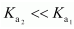for H2SO4 in water?

Solution :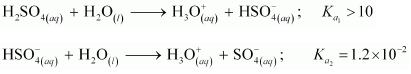It can be noticed that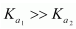This is because a neutral H2SO4 has a much higher tendency to lose a proton than the negatively charged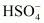. Thus, the former is a much stronger acid than the latter.

Question26.Considering the parameters such as bond dissociation enthalpy, electron gain enthalpy and hydration enthalpy, compare the oxidising power of F2 and Cl2.

Solution :
Fluorine is a much stronger oxidizing agent than chlorine. The oxidizing power depends on three factors.

1. Bond dissociation energy

2. Electron gain enthalpy

3. Hydration enthalpy

The electron gain enthalpy of chlorine is more negative than that of fluorine. However, the bond dissociation energy of fluorine is much lesser than that of chlorine. Also, because of its small size, the hydration energy of fluorine is much higher than that of chlorine. Therefore, the latter two factors more than compensate for the less negative electron gain enthalpy of fluorine. Thus, fluorine is a much stronger oxidizing agent than chlorine.

Question27. Give two examples to show the anomalous behaviour of fluorine.

Solution :
Anomalous behaviour of fluorine

(i) It forms only one oxoacid as compared to other halogens that form a number of oxoacids.

(ii) Ionisation enthalpy, electronegativity, and electrode potential of fluorine are much higher than expected.

28. Sea is the greatest source of some halogens. Comment.

Solution :
Sea water contains chlorides, bromides, and iodides of Na, K, Mg, and Ca. However, it primarily contains NaCl. The deposits of dried up sea beds contain sodium chloride and carnallite, KCl.MgCl2.6H2O. Marine life also contains iodine in their systems. For example, sea weeds contain upto 0.5% iodine as sodium iodide. Thus, sea is the greatest source of halogens.

Question29. Give the reason for bleaching action of Cl2.

Solution :
When chlorine reacts with water, it produces nascent oxygen. This nascent oxygen then combines with the coloured substances present in the organic matter to oxide them into colourless substances.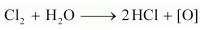Coloured substances + [O] → Oxidized colourless substance

Question30. Name two poisonous gases which can be prepared from chlorine gas.

Solution :
Two poisonous gases that can be prepared from chlorine gas are

(i) Phosgene (COCl2)

(ii) Mustard gas (ClCH2CH2SCH2CH2Cl)

Question31. Why is ICl more reactive than I2?

Solution :
ICl is more reactive than I2 because I−Cl bond in ICl is weaker than I−I bond in I2.

Question32. Why is helium used in diving apparatus?

Solution :
Air contains a large amount of nitrogen and the solubility of gases in liquids increases with increase in pressure. When sea divers dive deep into the sea, large amount of nitrogen dissolves in their blood. When they come back to the surface, solubility of nitrogen decreases and it separates from the blood and forms small air bubbles. This leads to a dangerous medical condition called bends. Therefore, air in oxygen cylinders used for diving is diluted with helium gas. This is done as He is sparingly less soluble in blood.

Question33. Balance the following equation: XeF6 + H2O → XeO2F2 + HF

Solution :
Balanced equation

XeF6 + 2 H2O → XeO2F2 + 4 HF

Question34. Why has it been difficult to study the chemistry of radon?

Solution :
It is difficult to study the chemistry of radon because it is a radioactive substance having a half-life of only 3.82 days. Also, compounds of radon such as RnF2 have not been isolated. They have only been identified.

Question35. Discuss the general characteristics of Group 15 elements with reference to their electronic configuration, oxidation state, atomic size, ionisation enthalpy and electronegativity.

Solution :
General trends in group 15 elements

(i) Electronic configuration: All the elements in group 15 have 5 valence electrons. Their general electronic configuration is ns2 np3.

(ii) Oxidation states: All these elements have 5 valence electrons and require three more electrons to complete their octets. However, gaining electrons is very difficult as the nucleus will have to attract three more electrons. This can take place only with nitrogen as it is the smallest in size and the distance between the nucleus and the valence shell is relatively small. The remaining elements of this group show a formal oxidation state of −3 in their covalent compounds. In addition to the −3 state, N and P also show −1 and −2 oxidation states.

All the elements present in this group show +3 and +5 oxidation states. However, the stability of +5 oxidation state decreases down a group, whereas the stability of +3 oxidation state increases. This happens because of the inert pair effect.

(iii) Ionization energy and electronegativity:

First ionization decreases on moving down a group. This is because of increasing atomic sizes. As we move down a group, electronegativity decreases, owing to an increase in size.

(iv) Atomic size: On moving down a group, the atomic size increases. This increase in the atomic size is attributed to an increase in the number of shells.

Question36. Why does the reactivity of nitrogen differ from phosphorus?

Solution :
Nitrogen is chemically less reactive. This is because of the high stability of its molecule, N2. In N2, the two nitrogen atoms form a triple bond. This triple bond has very high bond strength, which is very difficult to break. It is because of nitrogen’s small size that it is able to form pπ−pπ bonds with itself. This property is not exhibited by atoms such as phosphorus. Thus, phosphorus is more reactive than nitrogen.

Question37. Discuss the trends in chemical reactivity of group 15 elements.

Solution :
General trends in chemical properties of group − 15

(i) Reactivity towards hydrogen:

The elements of group 15 react with hydrogen to form hydrides of type EH3, where E = N, P, As, Sb, or Bi. The stability of hydrides decreases on moving down from NH3 to BiH3.

(ii) Reactivity towards oxygen:

The elements of group 15 form two types of oxides: E2O3 and E2O5, where E = N, P, As, Sb, or Bi. The oxide with the element in the higher oxidation state is more acidic than the other. However, the acidic character decreases on moving down a group.

(iii) Reactivity towards halogens:

The group 15 elements react with halogens to form two series of salts: EX3 and EX5. However, nitrogen does not form NX5 as it lacks the d-orbital. All trihalides (except NX3) are stable.

(iv) Reactivity towards metals:

The group 15 elements react with metals to form binary compounds in which metals exhibit −3 oxidation states.

Question38. Why does NH3 form hydrogen bond but PH3 does not?

Solution :
Nitrogen is highly electronegative as compared to phosphorus. This causes a greater attraction of electrons towards nitrogen in NH3 than towards phosphorus in PH3. Hence, the extent of hydrogen bonding in PH3 is very less as compared to NH3.

Question39. How is nitrogen prepared in the laboratory? Write the chemical equations of the reactions involved.

Solution :
An aqueous solution of ammonium chloride is treated with sodium nitrite.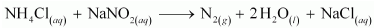NO and HNO3 are produced in small amounts. These are impurities that can be removed on passing nitrogen gas through aqueous sulphuric acid, containing potassium dichromate.

Question40. How is ammonia manufactured industrially?

Solution :
Ammonia is prepared on a large-scale by the Haber’s process.

N2(g) + 3H2(g) ⇋ 2NH3(g)   ΔfHo = −46.1 kJ/mol

The optimum conditions for manufacturing ammonia are:

(i) Pressure (around 200 × 105 Pa)

(ii) Temperature (700 K)

(iii) Catalyst such as iron oxide with small amounts of Al2O3 and K2O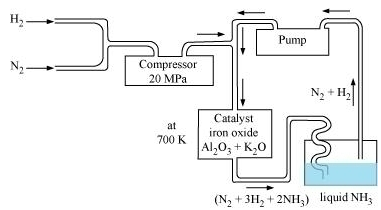Question41. Illustrate how copper metal can give different products on reaction with HNO3.

Solution :
Concentrated nitric acid is a strong oxidizing agent. It is used for oxidizing most metals. The products of oxidation depend on the concentration of the acid, temperature, and also on the material undergoing oxidation.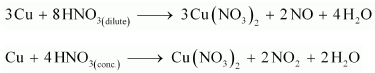Question42. Give the resonating structures of NO2 and N2O5.

Solution :
(1)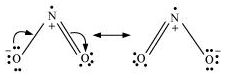(2)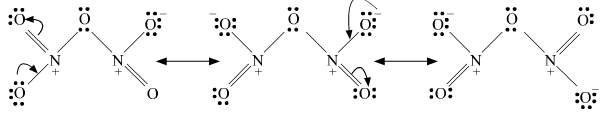Question43. The HNH angle value is higher than HPH, HAsH and HSbH angles. Why? [Hint: Can be explained on the basis of sp3 hybridisation in NH3 and only s−p bonding between hydrogen and other elements of the group].

Solution :
Hydride NH3 PH3 AsH3 SbH3

H−M−H angle 107° 92° 91° 90°

The above trend in the H−M−H bond angle can be explained on the basis of the electronegativity of the central atom. Since nitrogen is highly electronegative, there is high electron density around nitrogen. This causes greater repulsion between the electron pairs around nitrogen, resulting in maximum bond angle. We know that electronegativity decreases on moving down a group. Consequently, the repulsive interactions between the electron pairs decrease, thereby decreasing the H−M−H bond angle.

Question44. Why does R3P=O exist but R3N=O does not (R = alkyl group)?

Solution :
N (unlike P) lacks the d-orbital. This restricts nitrogen to expand its coordination number beyond four. Hence, R3N=O does not exist.

Question45. Explain why NH3 is basic while BiH3 is only feebly basic.

Solution :
NH3 is distinctly basic while BiH3 is feebly basic.

Nitrogen has a small size due to which the lone pair of electrons is concentrated in a small region. This means that the charge density per unit volume is high. On moving down a group, the size of the central atom increases and the charge gets distributed over a large area decreasing the electron density. Hence, the electron donating capacity of group 15 element hydrides decreases on moving down the group.

Question46. Nitrogen exists as diatomic molecule and phosphorus as P4. Why?

Solution :
Nitrogen owing to its small size has a tendency to form pπ−pπ multiple bonds with itself. Nitrogen thus forms a very stable diatomic molecule, N2. On moving down a group, the tendency to form pπ−pπ bonds decreases (because of the large size of heavier elements). Therefore, phosphorus (like other heavier metals) exists in the P4 state.

Question47. Write main differences between the properties of white phosphorus and red phosphorus.

Solution :

 White phosphorus Red Phosphorus It is a soft and waxy solid. It possesses a garlic smell. It is a hard and crystalline solid, without any smell. It is poisonous. It is non-poisonous. It is insoluble in water but soluble in carbon disulphide. It is insoluble in both water and carbon disulphide. It undergoes spontaneous combustion in air. It is relatively less reactive. In both solid and vapour states, it exists as a P4 molecule.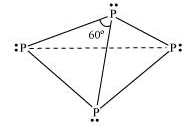It exists as a chain of tetrahedral P4 units.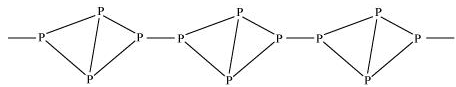Question49. Why does nitrogen show catenation properties less than phosphorus?

Solution :
Catenation is much more common in phosphorous compounds than in nitrogen compounds. This is because of the relative weakness of the N−N single bond as compared to the P−P single bond. Since nitrogen atom is smaller, there is greater repulsion of electron density of two nitrogen atoms, thereby weakening the N−N single bond.

Question50. Give the disproportionation reaction of H3PO3.

Solution :
On heating, orthophosphorus acid (H3PO3) disproportionates to give orthophosphoric acid (H3PO4) and phosphine (PH3). The oxidation states of P in various species involved in the reaction are mentioned below.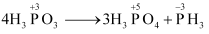Question51. Can PCl5 act as an oxidising as well as a reducing agent? Justify.

Solution :
PCl5 can only act as an oxidizing agent. The highest oxidation state that P can show is +5. In PCl5, phosphorus is in its highest oxidation state (+5). However, it can decrease its oxidation state and act as an oxidizing agent.

Question52. Justify the placement of O, S, Se, Te and Po in the same group of the periodic table in terms of electronic configuration, oxidation state and hydride formation.

Solution :
The elements of group 16 are collectively called chalcogens.

(i) Elements of group 16 have six valence electrons each. The general electronic configuration of these elements is ns2 np4, where n varies from 2 to 6.

(ii) Oxidation state:

As these elements have six valence electrons (ns2 np4), they should display an oxidation state of −2. However, only oxygen predominantly shows the oxidation state of −2 owing to its high electronegativity. It also exhibits the oxidation state of −1 (H2O2), zero (O2), and +2 (OF2). However, the stability of the −2 oxidation state decreases on moving down a group due to a decrease in the electronegativity of the elements. The heavier elements of the group show an oxidation state of +2, +4, and +6 due to the availability of d-orbitals.

(iii) Formation of hydrides:

These elements form hydrides of formula H2E, where E = O, S, Se, Te, PO. Oxygen and sulphur also form hydrides of type H2E2. These hydrides are quite volatile in nature.

Question53. Why is dioxygen a gas but sulphur a solid?

Solution :
Oxygen is smaller in size as compared to sulphur. Due to its smaller size, it can effectively form pπ−pπ bonds and form O2 (O==O) molecule. Also, the intermolecular forces in oxygen are weak van der Wall’s, which cause it to exist as gas. On the other hand, sulphur does not form M2 molecule but exists as a puckered structure held together by strong covalent bonds. Hence, it is a solid.

Question54. Knowing the electron gain enthalpy values for O → O and O → O2− as −141 and 702 kJ mol−1 respectively, how can you account for the formation of a large number of oxides having O2− species and not O? (Hint: Consider lattice energy factor in the formation of compounds).

Solution :
Stability of an ionic compound depends on its lattice energy. More the lattice energy of a compound, more stable it will be.

Lattice energy is directly proportional to the charge carried by an ion. When a metal combines with oxygen, the lattice energy of the oxide involving O2− ion is much more than the oxide involving O ion. Hence, the oxide having O2− ions are more stable than oxides having O. Hence, we can say that formation of O2− is energetically more favourable than formation of O.

Question55. Which aerosols deplete ozone?

Solution :
Freons or chlorofluorocarbons (CFCs) are aerosols that accelerate the depletion of ozone. In the presence of ultraviolet radiations, molecules of CFCs break down to form chlorine-free radicals that combine with ozone to form oxygen.

Question56. Describe the manufacture of H2SO4 by contact process?

Solution :
Sulphuric acid is manufactured by the contact process. It involves the following steps:

Step (i): Sulphur or sulphide ores are burnt in air to form SO2.

Step (ii): By a reaction with oxygen, SO2 is converted into SO3 in the presence of V2O5 as a catalyst.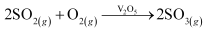Step (iii): SO3 produced is absorbed on H2SO4 to give H2S2O7 (oleum).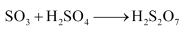This oleum is then diluted to obtain H2SO4 of the desired concentration.

In practice, the plant is operated at 2 bar (pressure) and 720 K (temperature). The sulphuric acid thus obtained is 96-98% pure.

Question57. How is SO2 an air pollutant?

Solution :
Sulphur dioxide causes harm to the environment in many ways:

• It combines with water vapour present in the atmosphere to form sulphuric acid. This causes acid rain. Acid rain damages soil, plants, and buildings, especially those made of marble.
• Even in very low concentrations, SO2 causes irritation in the respiratory tract. It causes throat and eye irritation and can also affect the larynx to cause breathlessness.
• It is extremely harmful to plants. Plants exposed to sulphur dioxide for a long time lose colour from their leaves. This condition is known as chlorosis. This happens because the formation of chlorophyll is affected by the presence of sulphur dioxide.

Question58. Why are halogens strong oxidising agents?

Solution :
The general electronic configuration of halogens is np5, where n = 2-6. Thus, halogens need only one more electron to complete their octet and to attain the stable noble gas configuration. Also, halogens are highly electronegative with low dissociation energies and high negative electron gain enthalpies. Therefore, they have a high tendency to gain an electron. Hence, they act as strong oxidizing agents.

Question59. Explain why fluorine forms only one oxoacid, HOF.

Solution :
Fluorine forms only one oxoacid i.e., HOF because of its high electronegativity and small size.

Question60. Explain why inspite of nearly the same electronegativity, oxygen forms hydrogen bonding while chlorine does not.

Solution :
Both chlorine and oxygen have almost the same electronegativity values, but chlorine rarely forms hydrogen bonding. This is because in comparison to chlorine, oxygen has a smaller size and as a result, a higher electron density per unit volume.

Question61. Write two uses of ClO2.

Solution :
Uses of ClO2:

• It is used for purifying water.
• It is used as a bleaching agent.

Question62. Why are halogens coloured?

Solution :
Almost all halogens are coloured. This is because halogens absorb radiations in the visible region. This results in the excitation of valence electrons to a higher energy region. Since the amount of energy required for excitation differs for each halogen, each halogen displays a different colour.

Question63. Write the reactions of F2 and Cl2 with water.

Solution :
(i)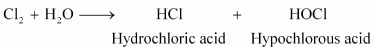(ii)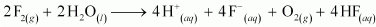Question64. How can you prepare Cl2 from HCl and HCl from Cl2? Write reactions only.

Solution :
(i) Cl2 can be prepared from HCl by Deacon’s process.(ii) HCl can be prepared from Cl2 on treating it with water.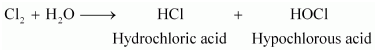Question65. What inspired N. Bartlett for carrying out reaction between Xe and PtF6?

Solution :
Neil Bartlett initially carried out a reaction between oxygen and PtF6. This resulted in the formation of a red compound,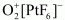.

Later, he realized that the first ionization energy of oxygen (1175 kJ/mol) and Xe (1170 kJ/mol) is almost the same. Thus, he tried to prepare a compound with Xe and PtF6. He was successful and a red-coloured compound,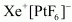was formed.

Question66. What are the oxidation states of phosphorus in the following:

(i) H3PO3 (ii) PCl3 (iii) Ca3P2

(iv) Na3PO4 (v) POF3?

Solution :
Let the oxidationn states of p be x

(i) H3PO3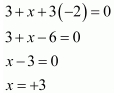(ii) PCl3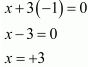(iii) Ca3P2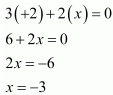(iv) Na3PO4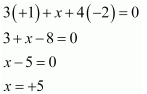(v) POF3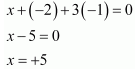Question67. Write balanced equations for the following:

(i) NaCl is heated with sulphuric acid in the presence of MnO2.

(ii) Chlorine gas is passed into a solution of NaI in water.

Solution :
(i)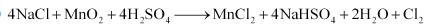(ii)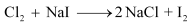Question68. How are xenon fluorides XeF2, XeF4 and XeF6 obtained?

Solution :
XeF2, XeF4, and XeF6 are obtained by a direct reaction between Xe and F2. The condition under which the reaction is carried out determines the product.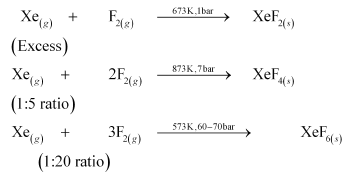Question69. With what neutral molecule is ClO isoelectronic? Is that molecule a Lewis base?

Solution :
ClOis isoelectronic to ClF. Also, both species contain 26 electrons in all as shown.

Total electrons ClO = 17 + 8 + 1 = 26

In ClF = 17 + 9 = 26

ClF acts like a Lewis base as it accepts electrons from F to form ClF3.

Question70. How are XeO3 and XeOF4 prepared?

Solution :
(i) XeO3 can be prepared in two ways as shown.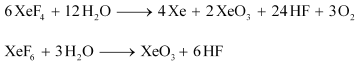(ii) XeOF4 can be prepared using XeF6.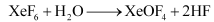Question71. Arrange the following in the order of property indicated for each set:

(i) F2, Cl2, Br2, I2 - increasing bond dissociation enthalpy.

(ii) HF, HCl, HBr, HI - increasing acid strength.

(iii) NH3, PH3, AsH3, SbH3, BiH3 − increasing base strength.

Solution :
(i) Bond dissociation energy usually decreases on moving down a group as the atomic size increases. However, the bond dissociation energy of F2 is lower than that of Cl2 and Br2. This is due to the small atomic size of fluorine. Thus, the increasing order for bond dissociation energy among halogens is as follows:

I2 < F2 < Br2 < Cl2

(ii) HF < HCl < HBr < HI

The bond dissociation energy of H-X molecules where X = F, Cl, Br, I, decreases with an increase in the atomic size. Since H-I bond is the weakest, HI is the strongest acid.

(iii) BiH3 ≤ SbH3 < AsH3 < PH3 < NH3

On moving from nitrogen to bismuth, the size of the atom increases while the electron density on the atom decreases. Thus, the basic strength decreases.

Question72. Which one of the following does not exist?

(i) XeOF4 (ii) NeF2

(iii) XeF2 (iv) XeF6

Solution :
NeF2 does not exist.

Question73. Give the formula and describe the structure of a noble gas species which is isostructural with: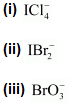Solution :
(i)

XeF4 is isoelectronic with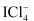and has square planar geometry.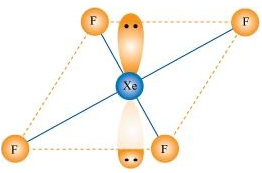(ii) XeF2 is isoelectronic to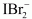and has a linear structure.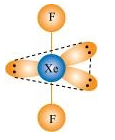(iii)  XeO3 is isostructural to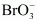and has a pyramidal molecular structure.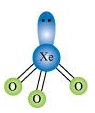Question74. Why do noble gases have comparatively large atomic sizes?

Solution :
Noble gases do not form molecules. In case of noble gases, the atomic radii corresponds to van der Waal’s radii. On the other hand, the atomic radii of other elements correspond to their covalent radii. By definition, van der Waal’s radii are larger than covalent radii. It is for this reason that noble gases are very large in size as compared to other atoms belonging to the same period.

Question75. List the uses of Neon and argon gases.

Solution :
Uses of neon gas:

• It is mixed with helium to protect electrical equipments from high voltage.
• It is filled in discharge tubes with characteristic colours.
• It is used in beacon lights.

Uses of Argon gas:

• Argon along with nitrogen is used in gas-filled electric lamps. This is because Ar is more inert than N.
• It is usually used to provide an inert temperature in a high metallurgical process.
• It is also used in laboratories to handle air-sensitive substances.